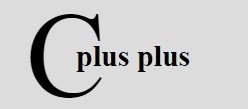# C++ Queue

A queue is a FIFO (First in First Out) structure and physically it can be implemented either as an array or as a linked list. Whatever way a queue is implemented, insertions take place at the "rear" end and deletions at the "front" end.

## Array Queue

When a queue is created as an array, its number of elements is declared before processing. The beginning of the array becomes its "front" end and the end of the array becomes its "rear" end. The terms "front" and "rear" are used in describing a linear list only when it is implemented as a queue.

"Front" stores the index of first element in the queue and "rear" stores the index of the last element in the queue. The number of elements in a queue at any given time can be calculated from the values of the "front" and the "rear".
If front = 0 then number of elements = 0
else number of elements = front - rear + 1.

## C++ Queue Example

Here are some example programs listed, demonstrating the concept of queue in C++ practically.

### Insertion in Array-Queue

```/* C++ Queue - Example Program of C++ Queue
* This program demonstrates the concept of
* insertion in an array queue in C++ */

#include<iostream.h>
#include<conio.h>
#include<stdlib.h>

int insert_in_queue(int [], int);
void display(int [], int, int);

const int SIZE = 50;

int queue[SIZE];
int front=-1;
int rear=-1;

void main()
{
clrscr();
int item, check;
char ch='y';

while(ch=='y' || ch=='Y')
{
cout<<"Enter item for insertion: ";
cin>>item;
check = insert_in_queue(queue, item);
if(check == -1)
{
cout<<"\nOverflow..!!..Aborting..!!..Press a key to exit..\n";
getch();
exit(1);
}
cout<<"Item inserted successfully..!!\n";
cout<<"\nNow the Queue (Front...to...Rear) is:\n";
display(queue, front, rear);
cout<<"\nWant to insert more ? (y/n).. ";
cin>>ch;
}
getch();
}

int insert_in_queue(int queue[], int elem)
{
if(rear == SIZE-1)
{
return -1;
}
else if(rear == -1)
{
front = rear = 0;
queue[rear] = elem;
}
else
{
rear++;
queue[rear] = elem;
}
return 0;
}

void display(int queue[], int front, int rear)
{
if(front == -1)
{
return;
}
for(int i=front; i<rear; i++)
{
cout<<queue[i]<<" <- ";
}
cout<<queue[rear]<<"\n";
}```

Here is the sample output of the above C++ program: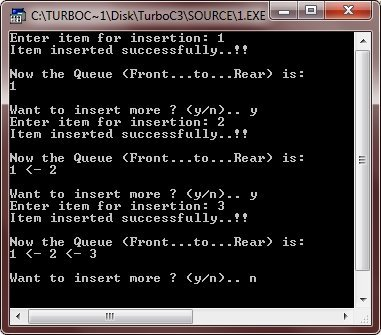### Deletion from Array-Queue

```/* C++ Queue - Example Program of C++ Queue
* This program demonstrates the concept of
* deletion in an array queue in C++ */

#include<iostream.h>
#include<conio.h>
#include<stdlib.h>

int delete_from_queue(int []);
int insert_in_queue(int [], int);
void display(int [], int, int);

const int SIZE = 50;

int queue[SIZE];
int front=-1;
int rear=-1;

void main()
{
clrscr();
int item, check;
char ch='y';

while(ch=='y' || ch=='Y')
{
cout<<"Enter item for insertion: ";
cin>>item;
check = insert_in_queue(queue, item);
if(check == -1)
{
cout<<"\nOverflow..!!..Aborting..!!..Press a key to exit..\n";
getch();
exit(1);
}
cout<<"Item inserted successfully..!!\n";
cout<<"\nNow the Queue (Front...to...Rear) is:\n";
display(queue, front, rear);
cout<<"\nWant to insert more ? (y/n).. ";
cin>>ch;
}

clrscr();
cout<<"Now deletion of elements starts...\n";
ch='y';
while(ch=='y' || ch=='Y')
{
check = delete_from_queue(queue);
if(check == -1)
{
cout<<"\nUnderflow..!!..Aborting..!!..Pres a key to exit..\n";
getch();
exit(2);
}
else
{
cout<<"\nElement deleted is: "<<check<<"\n";
cout<<"Now the Queue (Front...to...Rear) is:\n";
display(queue, front, rear);
}
cout<<"\nWant to delete more ? (y/n)... ";
cin>>ch;
}

getch();
}

int insert_in_queue(int queue[], int elem)
{
if(rear == SIZE-1)
{
return -1;
}
else if(rear == -1)
{
front = rear = 0;
queue[rear] = elem;
}
else
{
rear++;
queue[rear] = elem;
}
return 0;
}

int delete_from_queue(int queue[])
{
int retn;
if(front == -1)
{
return -1;
}
else
{
retn = queue[front];
if(front == rear)
{
front = rear = -1;
}
else
{
front++;
}
}
return retn;
}

void display(int queue[], int front, int rear)
{
if(front == -1)
{
return;
}
for(int i=front; i<rear; i++)
{
cout<<queue[i]<<" <- ";
}
cout<<queue[rear]<<"\n";
}```

Here are some sample runs of the above C++ program: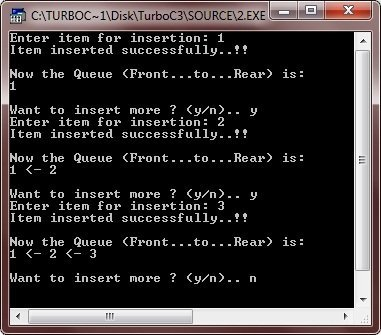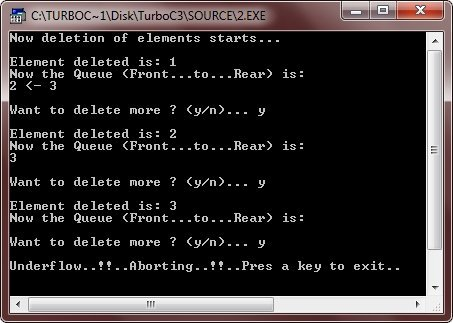## Linked Queue

Linked queue are the queues having links among its elements. Two pointers are maintained to store the "front" and the "rear" position.

### Insertion in Linked Queue

```/* C++ Queue - Example Program of C++ Queue
* This program demonstrates the concept of
* insertion in the linked queue in C++ */

#include<iostream.h>
#include<conio.h>
#include<stdlib.h>

struct node
{
int info;
node *next;
} *front, *newptr, *save, *ptr, *rear;

node *create_new_node(int);
void insert(node *);
void display(node *);

void main()
{
clrscr();
front = rear = NULL;
int inf;
int count=0;
char ch='y';

while(ch=='y' || ch=='Y')
{
cout<<"Enter information for the new node.. ";
cin>>inf;
newptr = create_new_node(inf);
if(newptr == NULL)
{
cout<<"\nSorry..!!..Cannot create new node..!!..Aborting..!!\n";
cout<<"Press any key to exit..\n";
getch();
exit(1);
}
insert(newptr);
cout<<"\nNow the Queue (Front...to...Rear) is:\n";
display(front);
cout<<"\nWant to enter more ? (y/n).. ";
cin>>ch;
}

getch();
}

node *create_new_node(int x)
{
ptr = new node;
ptr->info = x;
ptr->next = NULL;
return ptr;
}

void insert(node *n)
{
if(front == NULL)
{
front = rear = n;
}
else
{
rear->next = n;
rear = n;
}
}

void display(node *n)
{
while(n != NULL)
{
cout<<n->info<<" -> ";
n = n->next;
}
cout<<"!!\n";
}```

Here is the sample run of the above C++ program: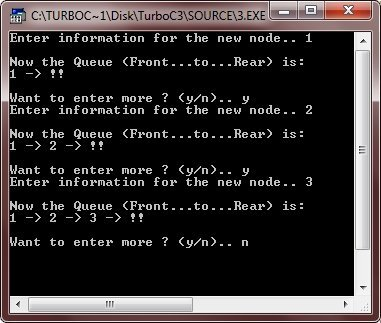### Deletion from Linked Queue

```/* C++ Queue - Example Program of C++ Queue
* This program demonstrates the concept of
* deletion from the linked queue in C++ */

#include<iostream.h>
#include<conio.h>
#include<stdlib.h>

struct node
{
int info;
node *next;
} *front, *newptr, *save, *ptr, *rear;

node *create_new_node(int);
void insert(node *);
void delete_node_queue();
void display(node *);

void main()
{
clrscr();
front = rear = NULL;
int inf;
int count=0;
char ch='y';

while(ch=='y' || ch=='Y')
{
cout<<"Enter information for the new node.. ";
cin>>inf;
newptr = create_new_node(inf);
if(newptr == NULL)
{
cout<<"\nSorry..!!..Cannot create new node..!!..Aborting..!!\n";
cout<<"Press any key to exit..\n";
getch();
exit(1);
}
insert(newptr);
cout<<"\nNow the Queue (Front...to...Rear) is:\n";
display(front);
cout<<"\nWant to enter more ? (y/n).. ";
cin>>ch;
}

clrscr();
do
{
cout<<"The Linked-Queue now is (Front...to...Rear) is:\n";
display(front);
if(count==0)
{
cout<<"\nWant to delete ? (y/n).. ";
count++;
}
else
{
cout<<"\nWant to delete more ? (y/n).. ";
}
cin>>ch;
if(ch=='y' || ch=='Y')
{
delete_node_queue();
}
cout<<"\n";
}while(ch=='y' || ch=='Y');

getch();
}

node *create_new_node(int x)
{
ptr = new node;
ptr->info = x;
ptr->next = NULL;
return ptr;
}

void insert(node *n)
{
if(front == NULL)
{
front = rear = n;
}
else
{
rear->next = n;
rear = n;
}
}

void delete_node_queue()
{
if(front == NULL)
{
cout<<"\nOverflow..!!..Press a key to exit..\n";
getch();
exit(2);
}
else
{
ptr = front;
front = front->next;
delete ptr;
}
}

void display(node *n)
{
while(n != NULL)
{
cout<<n->info<<" -> ";
n = n->next;
}
cout<<"!!\n";
}```

Here are some sample runs of this C++ program: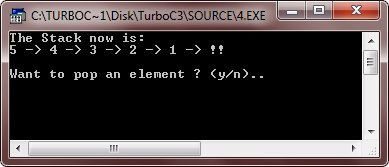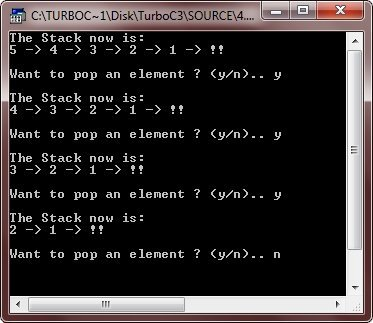Tools
Calculator

Quick Links
Signup - Login - Give Online Test